# New Bending Algorithm for Field-Driven Molecular Dynamics

## Abstract

A field-driven bending method is introduced in this paper according to the coordinate transformation between straight and curved coordinates. This novel method can incorporate with the periodic boundary conditions in analysis along axial, bending, and transverse directions. For the case of small bending, the bending strain can be compatible with the beam theory. Consequently, it can be regarded as a generalized SLLOD algorithm. In this work, the bulk copper beam under bending is analyzed first by the novel bending method. The bending stress estimated here is well consistent to the results predicted by the beam theory. Moreover, a hollow nanowire is also analyzed. The zigzag traces of atomic stress and the corresponding 422 common neighbor type can be observed near the inner surface of the hollow nanowire, which values are increased with an increase of time. It can be seen that the novel bending method with periodic boundary condition along axial direction can provide a more physical significance than the traditional method with fixed boundary condition.

## Introduction

The nano-scale mechanical properties become important since the size of electrical components is successively reduced for the portable convenience [1, 2]. Most of studies focused on mechanical properties related to the tension and compression. The problems of bending are actually met more frequently although it is composed by tension and compression. The bending tests of nanomaterials by using atomic simulation were widely applied. Liu et al.  simulated the pure bending of defect-free Al single crystals to investigate dislocation nucleation from free surfaces. They found that dislocation nucleation is not well represented by a critical value of the resolved shear stress but is reasonably well represented by the critical stress-gradient criterion. On the other hand, the size effects were also discussed widely. Miller and Shenoy  found that the surface elastic constant is the same order as the bulk elastic constant. The surface effect was also discussed in the bending case.

Unlike the case of tensile or compression tests of nanowire (NW) or nanofilm (NF) where the periodic boundary conditions (PBCs) were applied along the axial direction to remove the size effect, almost all the bending simulations took the ends of nanowire or nanofilm as fixed boundary conditions (FxBCs) . The FxBC is essentially inducing the size effects into the simulated objects. From the viewpoint of thermodynamics, the fixed atoms are viewed as zero velocities, and, thus, zero temperature at the fixed ends. In other words, all thermodynamic variables involving atom velocities are not well defined at the fixed boundary.

For the purpose of the computational efficiency, there are many methods to improve the computational speed. One of the methods is to use the synthetic system instead of real system. For example, Nose–Hoover algorithm , the synthetic thermostat variable generates the NVT ensemble more stably and efficiently than the rescaled velocity method , the latter cannot generate the NVT ensemble exactly. Moreover, the synthetic system usually combines the physical response into the equations of motion, thus can prevent the discontinuous trajectory of atoms and save the time to do the local equilibrium.

Non-equilibrium molecular dynamics (NEMD) can be described as two representations . One is the boundary-driven (BD) representation, the other is the field-driven (FD) representation. The FD method is belonging to the synthetic system. The BD method was used to calculate the thermal transport coefficients while the FD method was used to calculate the mechanical ones. One can mathematically transform the non-equilibrium boundary conditions for a thermal transport process into a mechanical field. The two representations of the system are said to be “congruent”. Almost all FD methods can combine with PBC while BD methods usually combine with FxBCs. In addition, since the non-equilibrium response is reflected in the equations of motion for FD method, it is no need to use the stepwise equilibrium-non-equilibrium cyclic driven that usually used in the BD method. Thus the FD method can be more efficient than BD method.

From the above reasons, a novel-bending algorithm is proposed and investigated in this paper. Based on the coordinate transformation from flat coordinate to curved one, the straight material is transformed to the curved one. The method belongs to FD method, and can be viewed as the generalized SLLOD algorithm . It also removes the fixed atoms generally used at FxBCs so that all thermodynamic variables involving atom velocities can be defined everywhere. The method for the bending algorithm is introduced in “Methodology” section. “Numerical Tests” section shows some numerical tests for both macroscopic and microscopic systems. Finally, it is concluded in “Conclusion” section.

## Methodology

### The Coordinate Transformation

For a tension simulation, one may view the stretch as a coordinate transformation described as(1)

The Jacobian and inverse Jacobian are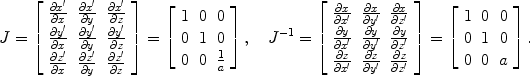(2)

From Fig. 1, the original straight material that has length L in the (x,y,z) coordinate transforms to (x,y,z′) coordinate. In the view of (x,y,z′) coordinate, the straight material has a length of aL. The factor 1/a of the transformation can be viewed as the factor of stretch. One can set the coordinate of next time (x(t),y(t),z(t)) to be equal to the coordinate (x,y,z′), thus the dynamical, uniform, and field-driven stretch can be performed.

From the above idea, one can search a coordinate transformation for the bending purpose. For the simplest bending case, one may consider the curved axis x′ as a quadratic curve of the form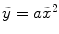(see Fig. 2). The slope at x′ can be obtained by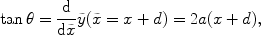(3)

where d is a horizontal distance between x and x′.

In order to satisfy the assumption of “a plane normal to the axis remains a plane normal to the curved axis after bending” in the beam theory , the y′ axis is set to be normal to the curved axis x′. Thus for an arbitrary point P(x,y) = P(x′,y′), the coordinate transformation can be obtained and given by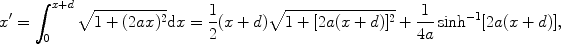(4)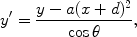(5)(6)

The distance d can be evaluated by the assumption that the normal at x′ is orthogonal to the tangent at x′, i.e.,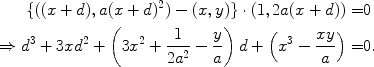(7)

It is a cubic equation having three roots. The discriminant D can be used to check the roots ,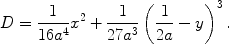(8)

For D < 0, there are three different real roots; for D = 0, there are triple or double real roots; and for D > 0, there is only one real root and two imaginary roots. The geometrical condition requests that the distance d is a real root, thus D must be greater than zero. One can see that if we set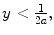then D must be greater than zero. Thus the condition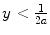is selected as a limit range of the coordinate transformation.

For a very small deflection, i.e.,the Eqs. 3–7can be reduced as(9)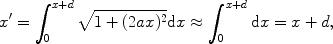(10)(11)(12)(13)

Thus the coordinate transformation and inverse transformation can be obtained,(14)

And the corresponding Jacobian and inverse Jacobian are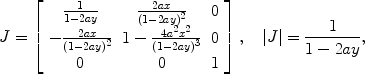(15)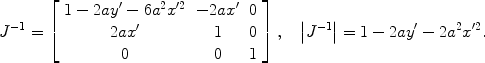(16)

It can be seen thatAfter constructing the J and J−1, the bending simulation can be performed as Fig. 3. The coordinate (x,y,z) is transformed to (x′,y′,z′) with the curvature-related coefficient a. Thus the simulated system is curved in the viewpoint of (x′,y′,z′) coordinate. The (x′,y′,z′) can then be taken as the coordinate at next time step to perform the bending dynamic simulation.

### The Bending Strain

For the curvature, it can be shown that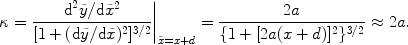(17)

Note that the curvature is independent of coordinates; it is convenient to characterize the bending status. For the displacement u x = x′x = d and u y = y′y = –a(x + d)2, the strain components can be obtained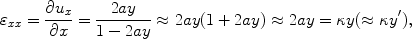(18)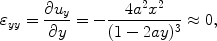(19)(20)

And the volume change can be calculated as(21)

where L,b, and c are the length, width, and depth of the beam, respectively. Thus the volume of the beam can be viewed as no change after bending. At y = 0, the axial strain ε xx = 0, thus the axis x′ can be considered as centroid axis or neutral surface. The linear relation between ε xx and y is also consistent with the assumption of beam theory . For ε xy = 0, it is also conformed to the assumption that plane sections initially normal to the beam axis remain plane and normal to that axis after bending. The transverse strain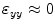also meets the assumption of beam theory. Thus the model can be used to verify the suitability of beam theory in the nanoscale model with slight bending.

### The SLOOD Algorithm

Let a(t) be a function of time t, the rate of displacement gradient tensor can be written as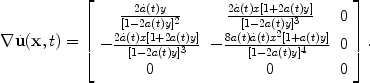(22)

During the motion of bending, a particle i can experience a velocity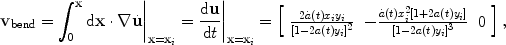(23)

where x i is the position of particle i. Thus the equations of motion for the position of atom i can be written as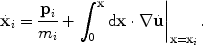(24)

By using the mean value theorem for vector-valued functions , the above equation can be rewritten as(25)

where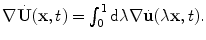Assuming the equation of motion for the conjugate momentum induced by the bending can be written as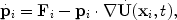(26)

where p i , F i , and m i are the conjugate momentum, force, and mass, respectively, of particle i. Equations 25 and 26 are one example of the general NEMD equations of motion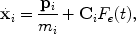(27)(28)

where C i and D i are the phase variables coupling of the field F e (t) to the system. Equations 25 and 26 can be reduced to original SLLOD algorithm if the rate of displacement gradient tensor is independent of coordinate . Thus Eqs. 25 and 26 can be viewed as the generalized SLLOD algorithm. Note that Eqs. 25 and 26 can also be used in the case with large deformation.

### Periodic Boundary Conditions

Since the curved coordinate and flat coordinate can be transformed to each other at each time step, one can transform the curved system to rectangular one, and then the PBCs can be applied (see Fig. 4). After the PBCs are applied, the atomic positions are restored to the curved coordinates and do the simulation at next time step.

The minimum image criteria (MIC) can also be implemented. As shown in Fig. 4, the curved cell can be transformed to rectangular one by J−1. After building the image cells, the neighbor list can be built up , and then the rectangular cell is restored to the curved cell by J. The forces between any neighbor atoms thus are calculated according to the neighbor list and the curved positions.

In the mathematical description of the primary cell, one can consider the position of atom i that is changed due to the bending essentially, i.e., the second term of Eq. 25. It is convenient to use a shape matrix h(x,t) to describe the primary cell so that x i (t) = s i h(x i ,t), where s i is the scaled position of atom i. Thus the position x i is fully changed by h if the scaled position s i is not a function of t, and the second term of Eq. 25 becomes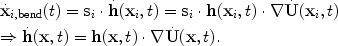(29)

Equation 29 then serves the equation of motion for the primary cell.

## Numerical Tests

### Bulk Copper Beam

The bulk bending simulation is first tested for verifying the new bending method. The copper atomic system is constructed as 20aLattice × 10aLattice × 10aLattice(equals to 72.38 × 36.19 × 36.19 Å3) with 8000 atoms, where aLattice = 3.619 Å is the lattice constant for copper. The x-,y-, and z-directions are along to the , , and  orientations, respectively. The PBCs/MIC are imposed along the x-,y-, and z- directions, and the Morse potential is adopted . Verlet neighbor list combined with cell-link method is used . Gesar fifth-order predictor–corrector algorithm  with time step 1 fs is also applied. The atomic stress formula for the atomic system is calculated by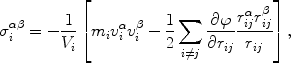(30)

whereis the atomic stress with component αβ for atom i; m iare the mass for atom i α component velocity for atom i, α component distance between atoms i and j, respectively; and ϕ is the potential energy. The total stress for the whole system is(31)

where the total volume V and atomic volume V i are related by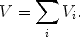(32)

The system is equilibrated first in the NσT ensemble with GGMT thermostat method (with five thermostat variables)  at 300 K and MTK barostat method  at 0 GPa during 0.1 ns. Then the system is bended with bending rate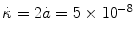fs−1 Å−1, which equals to the bending rate at the beam end of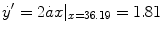rad ns−1, and the temperature is controlled at 300 K with GGMT method.

The front and isometric views of the bending system with curvature κ = 4.5 × 10−3 Å−1 are shown in Fig. 5. According to the beam theory , the normal stress σ xx can be estimated by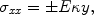(33)

where E is the Young’s modulus. For Cu, E = 117.2 GPa , thus the maximum and minimum stresses are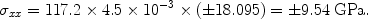(34)

The simulated values with the bending algorithm are σmax = 7.81 GPa and σmin = −6.79 GPa which take the average among the top and bottom atomic layers, respectively. The values of atomic simulation are close to the one estimated by continuous mechanical beam theory.

If the crystalline effect is considered in the Young’s modulus which is given by(35)

where C11C12, and C44 are the elastic moduli, (n x ,n y ,n z ) is the crystalline orientation. For Cu, the moduli are C11 = 168.4 GPa, C12 = 121.4 GPa, and C44 = 75.4 GPa, respectively, at 300 K . Thus the Young’s modulus along  orientation is E = 66.69 GPa, and gives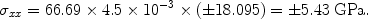(36)

The values of the axial stress obtained in this work are distributed just in between. The possible cause is that the lattice constant is stretched/compressed so that the elastic moduli are no longer the same as reference . Thus the axial stresses at top and bottom are not symmetric, and deviate from Eq. 36.

Once the model is compatible with macroscopic bulk, it is confident to use the bending method to simulate the microscopic nano-system.

### Hollow Copper Nanowire

Nanowires (NWs) exhibit an interesting quantum conductance behavior even at room temperature. Electron transport properties for NWs have been investigated extensively due to their significant importance in a variety of applications . Diao et al.  investigated the elastic properties of Au NWs by molecular statics, and found that due to the surface effects, the smaller the cross-sectional area the higher the Young’s modulus in the NWs without undergoing the phase transformation. Chen and Chen  studied the Au NWs subjected to uniaxial tension at high strain-rate under different temperatures. They found the microstructures of NWs were transformed first from FCC to face-centred-orthorhombic-like crystalline, and then changed to the amorphous state. Moreover, it was predicted that the conductance at high strain-rate deformation may be no longer quantized. Recent research has revealed that geometry, including surface orientation and the hollowness of nanomaterials, can also greatly affect their behavior .

The works of Jiang and Zheng [27, 28] are referred here to compare the effect induced by different boundaries. The system size studied in this paper is same as Zheng’s work (outer and inner cross-section parameters are 10aLattice and 4alattice, respectively), except the PBC is applied here along the axial direction instead of FxBC used in Zheng’s work. Other settings remain the same as previous sub-section. The system is equilibrated in NVT ensemble with GGMT method at 10 K before bending.

The axial stress distribution of hollow NW after bending with κ = 2.25 × 10−3 Å−1 at 10 K is shown in Fig. 6. It is observed that higher tensile stresses are created near the corner and dislocations. Higher stress at corner is mainly induced by the surface tension; while the dislocations are found at (100) surfaces, identical to report in the reference .

The technique of common neighbor analysis (CNA) is adopted to analyze the local structure distribution [23, 29, 30]. The CNA has three indices, j k, and l, which denote the number of common neighbor (CN) particles, the pair number of CN particles, and the number of CN pairs that makes up a chain, respectively. A pair is constructed by two particles whose distance apart is less than a cutoff radius. The cutoff radius is chosen to be 1.2dNN, where dNN is the nearest-neighbor distance. For the perfect FCC structure, the probability of 421 CN type is 100%, while the perfect HCP structure contains 50% 421 CN type and 50% 422 CN type. The structures of 421 and 422 CN types are shown in Fig. 7 for reference.

Figure 8 shows the distributions of 422 CN type and stress at cross-section of the hollow NW at different time. The upper pictures in Fig. 8 show the 422 CN-type distributions. The lower pictures show the axial stress σ xx distributions with color range from −10 to 10 GPa. The growing atomic tensile stress can be observed, and the 422 CN-type growths are accompanied with. The stress trace and 422 CN type initially grow alongdirection, and reflected to  direction by the inner corner, then form a zigzag trace. Note that the depth of the stress trace and 422 CN type is only about one or two atomic layers from the inner surface.

Since the PBC is applied here, the atoms at the boundary are movable so that the stress trace can grow through the ends. On the contrary, if the FxBC is applied, the atoms at the ends will be fixed, and the interface between movable and fixed atoms will lead to an artificially induced crack, obviously violating the physical phenomenon.

## Conclusion

In this study, the synthetic, field-driven bending method is introduced by using the coordinate transformation between straight and curved coordinates. The new method can incorporate with PBCs along axial, bending, and transverse directions. For problem with small bending effect, the bending strains evaluated by this method are well consistent with those predicted by the beam theory. Furthermore, it can be regarded as the generalized SLLOD algorithm. The accuracy and reliability of this novel bending method are verified by two examples, which are the bulk copper beam and the hollow NW under bending, respectively. The bending stress of the bulk copper beam estimated here is quite close to those predicted by the beam theory; while the atomic stress and the corresponding microstructure of 422 CN type near the inner surface of the hollow NW are increased with an increase of time. These results are well consistent with the earlier work. Moreover, the performance of this novel bending method can be significantly enhanced by using PBC along axial direction in the bending model by eliminating the artificial crack which is easily created by using traditional method with FxBC.

## References

1. Jian SR, Teng IJ, Yang PF, Lai YS, Lu JM, Chang JG, Ju SP: Nanoscale Res. Lett.. 2008, 3: 186. COI number [1:CAS:528:DC%2BD1cXhsVyhtr%2FK]; Bibcode number [2008NRL.....3..186J] 10.1007/s11671-008-9134-4

2. Lin YH, Jian SR, Lai YS, Yang PF: Nanoscale Res. Lett.. 2008, 3: 71. Bibcode number [2008NRL.....3...71L] Bibcode number [2008NRL.....3...71L] 10.1007/s11671-008-9119-3

3. Liu YF, van der Giessen E, Needleman A: Int. J. Solids Struct.. 2007, 44: 1719. COI number [1:CAS:528:DC%2BD2sXjs1Wms7w%3D] 10.1016/j.ijsolstr.2006.07.017

4. Miller RE, Shenoy VB: Nanotechnology. 2000, 11: 139. COI number [1:CAS:528:DC%2BD3cXnsVekt74%3D]; Bibcode number [2000Nanot..11..139M] 10.1088/0957-4484/11/3/301

5. Nosé S, Klein ML: Mol. Phys.. 1983, 50: 1055. Bibcode number [1983MolPh..50.1055N] Bibcode number [1983MolPh..50.1055N] 10.1080/00268978300102851

6. Nosé S: J. Chem. Phys.. 1984, 81: 511. Bibcode number [1984JChPh..81..511N] Bibcode number [1984JChPh..81..511N] 10.1063/1.447334

7. Hoover WG: Phys. Rev. A. 1985, 31: 1695. Bibcode number [1985PhRvA..31.1695H] Bibcode number [1985PhRvA..31.1695H] 10.1103/PhysRevA.31.1695

8. Haile JM: Molecular Dynamics Simulation: Elementary Methods. Wiley, New York; 1992.

9. Evans DJ, Morriss GP: Statistical Mechanics of Nonequilibrium Liquids. Academic press, San Diego; 1990.

10. Ugural AC: Mechanics of Materials. McGraw-Hill, Singapore; 1993.

11. James RC: Mathematics Dictionary. Springer, New York; 1992.

12. Flett TM: Tohoku Math. J.. 1972, 24: 141. 10.2748/tmj/1178241526

13. Ryckaert JP, Bellemans A, Ciccotti G, Paolini GV: Phys. Rev. A. 1989, 39: 259. Bibcode number [1989PhRvA..39..259R] Bibcode number [1989PhRvA..39..259R] 10.1103/PhysRevA.39.259

14. Rapaport DC: The Art of Molecular Dynamics Simulation. Cambridge University Press, Cambridge; 1995.

15. Basinski ZS, Duesbery MS, Taylor R: Can. J. Phys.. 1971, 49: 2160. COI number [1:CAS:528:DyaE3MXlsFGkt78%3D]; Bibcode number [1971CaJPh..49.2160B] 10.1139/p71-262

16. Liu Y, Tuckerman ME: J. Chem. Phys.. 2000, 112: 1685. COI number [1:CAS:528:DC%2BD3cXksVGgtA%3D%3D]; Bibcode number [2000JChPh.112.1685L] 10.1063/1.480769

17. Martyna GJ, Tobias DJ, Klein ML: J. Chem. Phys.. 1994, 101: 4177. COI number [1:CAS:528:DyaK2cXmtFeht7o%3D]; Bibcode number [1994JChPh.101.4177M] 10.1063/1.467468

18. Gray DE: American Institute of Physics Handbook. McGraw-Hill, New York; 1972.

19. Landau LD, Lifshitz EM: Theory of Elasticity. Pergamon, New York; 1986.

20. Kittel C: Introduction to Solid State Physics. Wiley, New York; 1996.

21. Brandbyge M, Schiøtz J, Sørensen MR, Stoltze P, Jacobsen KW, Nørskov JK, Olesen L, Laegsgaard E, Stensgaard I, Besenbacher F: Phys. Rev. B. 1995, 52: 8499. Bibcode number [1995PhRvB..52.8499B] Bibcode number [1995PhRvB..52.8499B] 10.1103/PhysRevB.52.8499

22. Diao J, Gall K, Dunn ML: J. Mech. Phys. Solids. 2004, 52: 1935. COI number [1:CAS:528:DC%2BD2cXmslyqtr4%3D]; Bibcode number [2004JMPSo..52.1935D] 10.1016/j.jmps.2004.03.009

23. Chen DL, Chen TC: Nanotechnology. 2005, 16: 2972. COI number [1:CAS:528:DC%2BD28XnvVKjsQ%3D%3D]; Bibcode number [2005Nanot..16.2972C] 10.1088/0957-4484/16/12/041

24. Sun YG, Mayers B, Herricks T, Xia YN: Nano Lett.. 2003, 3: 955. COI number [1:CAS:528:DC%2BD3sXksVSjtrc%3D]; Bibcode number [2003NanoL...3..955S] 10.1021/nl034312m

25. Jia CJ, Sun LD, Yan ZG, Pang YC, You LP, Yan CH: J. Phys. Chem. C. 2007, 111: 13022. COI number [1:CAS:528:DC%2BD2sXoslartr8%3D] 10.1021/jp073837q

26. Ji CJ, Park HS: Appl. Phys. Lett.. 2006, 89: 181916. Bibcode number [2006ApPhL..89r1916J] Bibcode number [2006ApPhL..89r1916J] 10.1063/1.2372748

27. Jiang S, Zhang HW, Zheng YG, Chen Z: J. Phys. D Appl. Phys.. 2009, 42: 135408. Bibcode number [2009JPhD...42m5408J] Bibcode number [2009JPhD...42m5408J] 10.1088/0022-3727/42/13/135408

28. Zheng YG, Zhang HW, Chen Z, Wang L, Zhang ZQ, Wan JB: Appl. Phys. Lett.. 2008, 92: 041913. Bibcode number [2008ApPhL..92d1913Z] Bibcode number [2008ApPhL..92d1913Z] 10.1063/1.2839581

29. Clarke AS, Jónsson H: Phys. Rev. E. 1993, 47: 3975. COI number [1:CAS:528:DyaK3sXltVemur4%3D]; Bibcode number [1993PhRvE..47.3975C] 10.1103/PhysRevE.47.3975

30. Faken D, Jónsson H: Comput. Mater. Sci.. 1994, 2: 279. COI number [1:CAS:528:DyaK2cXkvV2js7c%3D] 10.1016/0927-0256(94)90109-0

## Author information

Authors

### Corresponding author

Correspondence to Dao-Long Chen.

## Rights and permissions

Reprints and Permissions

Chen, DL., Chen, TC. & Lai, YS. New Bending Algorithm for Field-Driven Molecular Dynamics. Nanoscale Res Lett 5, 315 (2010). https://doi.org/10.1007/s11671-009-9482-8

• Accepted:

• Published:

• DOI: https://doi.org/10.1007/s11671-009-9482-8

### Keywords

• Molecular dynamics
• Field-driven
• Hollow nanowire
• Bending
• SLLOD algorithm